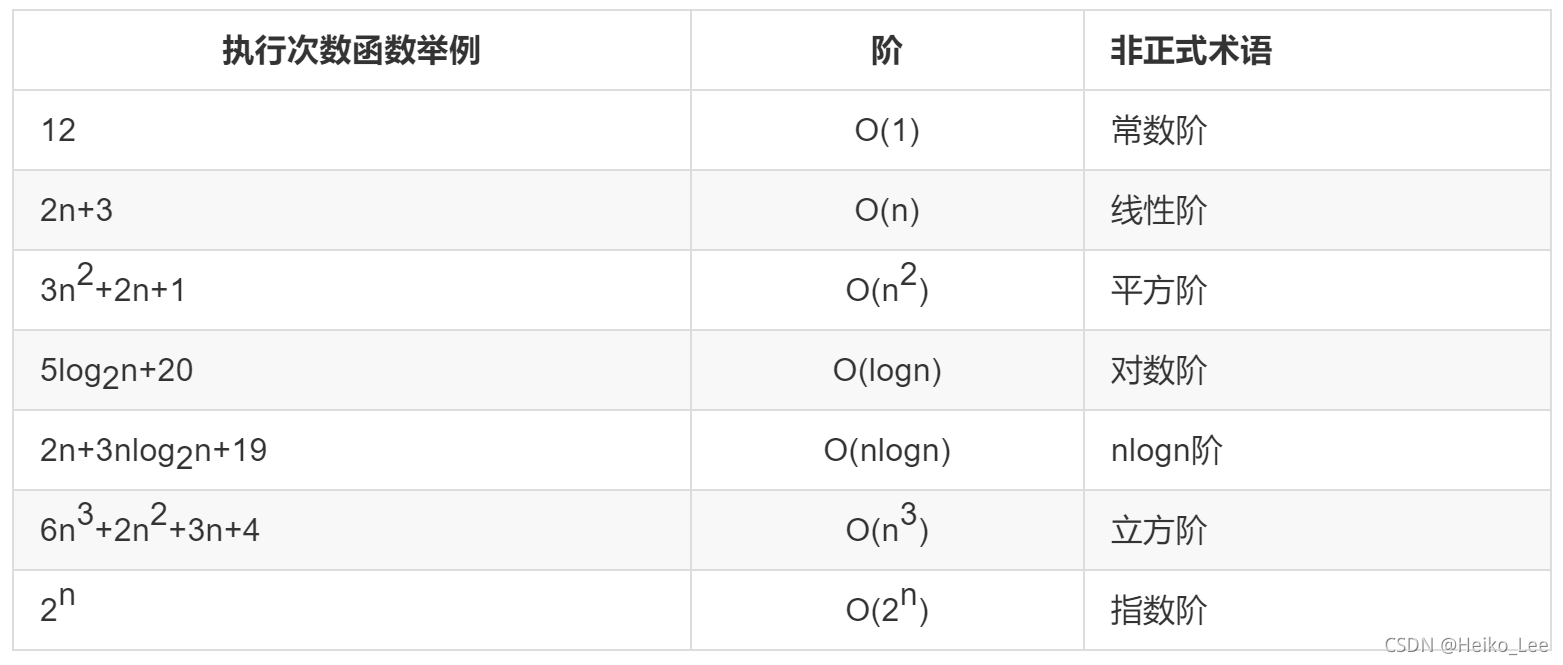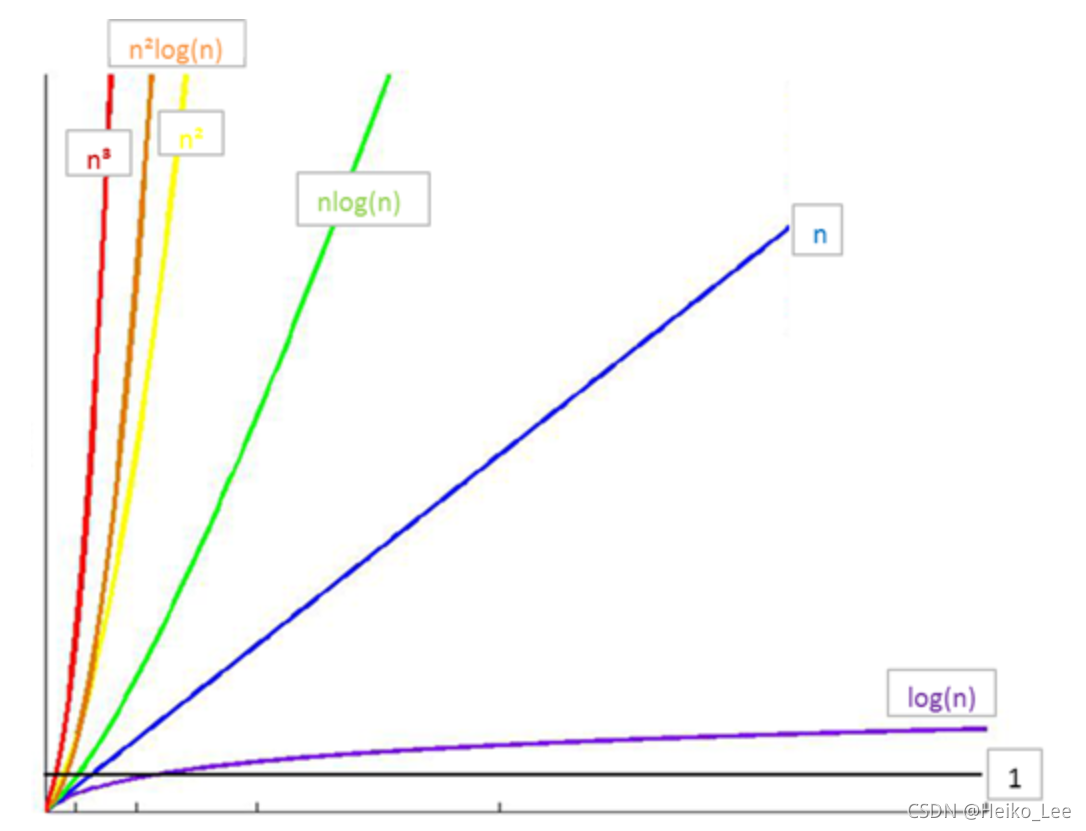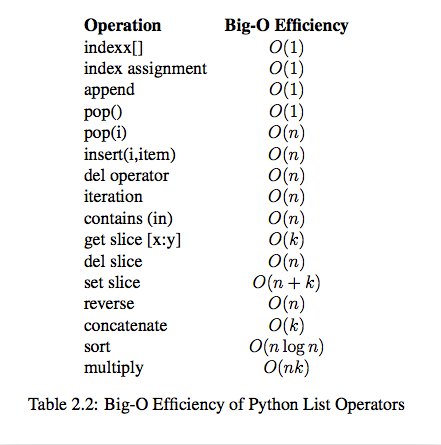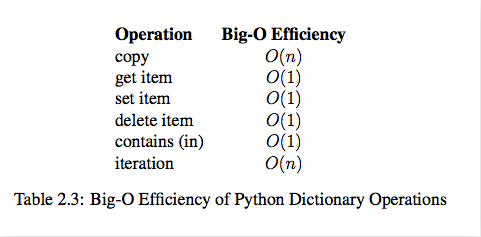# 数据结构与算法012234-川同学

, ,

# 1 引言

## 1.1 一个例子

for a in range(0, 1001):
for b in range(0, 1001):
for c in range(0, 1001):
if a**2 + b**2 == c**2 and a+b+c == 1000:
print("a, b, c: %d, %d, %d" % (a, b, c))


# 两重循环，知道了a和b，c就固定了
for a in range(0, 1001):
for b in range(0, 1001-a):
c = 1000 - a - b
if a**2 + b**2 == c**2:
print("a, b, c: %d, %d, %d" % (a, b, c))


## 1.2 算法的概念

1.输入: 算法具有0个或多个输入
2.输出: 算法至少有1个或多个输出
3.有穷性: 算法在有限的步骤之后会自动结束而不会无限循环，并且每一个步骤可以在可接受的时间内完成
4.确定性：算法中的每一步都有确定的含义，不会出现二义性
5.可行性：算法的每一步都是可行的，也就是说每一步都能够执行有限的次数完成

1.1中的两个方法都是算法，那如何衡量这俩个算法的好坏呢？

## 1.3 算法的衡量

“大O记法”：对于单调的整数函数f，如果存在一个整数函数g和实常数c>0，使得对于充分大的n总有f(n)<=c*g(n)，就说函数g是f的一个渐近函数（忽略常数），记为f(n)=O(g(n))。也就是说，在趋向无穷的极限意义下，函数f的增长速度受到函数g的约束，亦即函数f与函数g的特征相似。

## 1.4 计算时间复杂度

• 算法完成工作最少需要多少基本操作，即最优时间复杂度
• 算法完成工作最多需要多少基本操作，即最坏时间复杂度
• 算法完成工作平均需要多少基本操作，即平均时间复杂度
最优时间复杂度，反映的只是最乐观最理想的情况，没有参考价值。对于平均情况的计算，也会因为应用算法的实例分布可能并不均匀而难以计算。
因此，我们主要关注算法的最坏情况，亦即最坏时间复杂度。

• 基本操作，即只有常数项，认为其时间复杂度为O(1)
• 顺序结构，时间复杂度按加法进行计算
• 循环结构，时间复杂度按乘法进行计算
• 分支结构，时间复杂度取最大值
• 判断一个算法的效率时，往往只需要关注操作数量的最高次项，其它次要项和常数项可以忽略
• 在没有特殊说明时，我们所分析的算法的时间复杂度都是指最坏时间复杂度

## 1.5 常见时间复杂度O(1) < O(logn) < O(n) < O(nlogn) < O(n^2) < O(n^2logn) < O(n^3) < O(2^n) < O(n!) < O(n^n)

## 1.6 Python内置类型性能分析## 1.7 数据结构

### 1.7.1 概念

Python给我们提供了很多现成的数据结构类型，这些系统自己定义好的，不需要我们自己去定义的数据结构叫做Python的内置数据结构，比如列表、元组、字典。而有些数据组织方式，Python系统里面没有直接定义，需要我们自己去定义实现这些数据的组织方式，这些数据组织方式称之为Python的扩展数据结构，比如栈，队列等。

• 插入
• 删除
• 修改
• 查找
• 排序

### 评论 抢沙发### 觉得文章有用就打赏一下文章作者

#### 支付宝扫一扫打赏#### 微信扫一扫打赏Vieu3.3主题Q Q 登 录